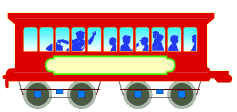Ch 7. Inverse Functions Multimedia Engineering Math InverseFunctions ExponentialFunctions Inverse Trig.Functions
 Chapter 1. Limits 2. Derivatives I 3. Derivatives II 4. Mean Value 5. Curve Sketching 6. Integrals 7. Inverse Functions 8. Integration Tech. 9. Integrate App. 10. Parametric Eqs. 11. Polar Coord. 12. Series Appendix Basic Math Units Search eBooks Dynamics Fluids Math Mechanics Statics Thermodynamics Author(s): Hengzhong Wen Chean Chin Ngo Meirong Huang Kurt Gramoll ©Kurt GramollMATHEMATICS - CASE STUDY IntroductionTony's Cart Tony is a driver of the Fastpass cart in the animal kingdom section of Funworld. He needs to drive the cart at a constant speed so that the customer can see the animals clearly. Before the cart reaches the constant speed, its velocity varies. What is known: The cart starts from rest. The velocity varies as a function of time, v = 2500 t2/3 mile/hour where t is in hours. The final constant speed of the cart is 10 mile/hour. Questions How long does it take the cart to reach the required speed? Approach When the velocity is positive, the function of speed is a one-to-one function.